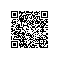# 8天玩转并行开发——第八天 用VS性能向导解剖你的程序

最后一篇，我们来说说vs的“性能向导"，通常我们调试程序的性能一般会使用Stopwatch,如果希望更加系统的了解程序，我们就需要

using System;
using System.Collections.Generic;
using System.Linq;
using System.Text;
using System.Diagnostics;

namespace Test
{
class Program
{
static object obj = new object();

static void Main(string[] args)
{
var watch = Stopwatch.StartNew();

var range = ParallelEnumerable.Range(1, 100000000);

var query = (from n in range.AsParallel()
where n % 5 == 0
select Calculate(n)).Average();

watch.Stop();

Debug.WriteLine("耗费时间:{0}", watch.Elapsed);
Console.WriteLine("耗费时间:{0}", watch.Elapsed);
}

/// <summary>
/// 模拟复杂的数学计算
/// </summary>
/// <param name="num"></param>
/// <returns></returns>
static double Calculate(int num)
{
lock (obj)
{
var sqrt = Math.Sqrt(num);

var pow = Math.Pow(sqrt, 5);

var log10 = Math.Log10(pow);

var floor = Math.Floor(log10);

return floor;
}
}
}
}①：从图中的绿色破浪线可以看出，我们的程序确实是多核计算。

②：并行计算耗时16515ms,平均CPU使用率：39%。，这里要注意，性能剖析器也需要耗费CPU周期,所以执行时间要稍大于实际时间。static double Calculate(int num)
{
var sqrt = Math.Sqrt(num);

var pow = Math.Pow(sqrt, 5);

var log10 = Math.Log10(pow);

var floor = Math.Floor(log10);

return floor;
}使用钉钉扫一扫加入圈子
+ 订阅